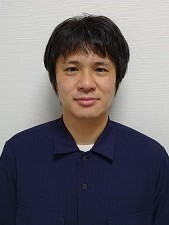# People

 Yukinobu Toda PositionProfessor (from 2017/07/16 ) show past_positionsResearch FieldMathematics Mathematics E-MailLast Update 2022/11/21

Algebraic varieties are geometric objects defined by zero locus of polynomials, and their properties have been studied for a long time in the form of such familiar shapes as parabolas and circles. On the other hand, a special class of algebraic varieties called Calabi-Yau manifolds is playing an important role in modern superstring theory, in which an interesting conjecture called mirror symmetry has been proposed. This conjecture claims that there should exist a symmetry between two different mathematical objects (algebraic varieties and symplectic manifolds), and now this conjecture is described by an abstract notion of triangulated categories. Triangulated categories corresponding to algebraic varieties are derived categories of coherent sheaves, and I have been studying derived categories.

My recent works are descriptions of the spaces of stability conditions on certain derived categories, and constructions of the moduli spaces of (semi) stable objects on them. Here the notion of stability conditions is considered to give a mathematical framework of BPS-branes in superstring theory, and we can observe several interesting symmetries other than mirror symmetry by studying derived categories and stability conditions on them. I believe that we can understand such symmetries uniformly, by establishing a new geometry based on derived categories, and developing the theory of stability conditions.

Back to Member List.